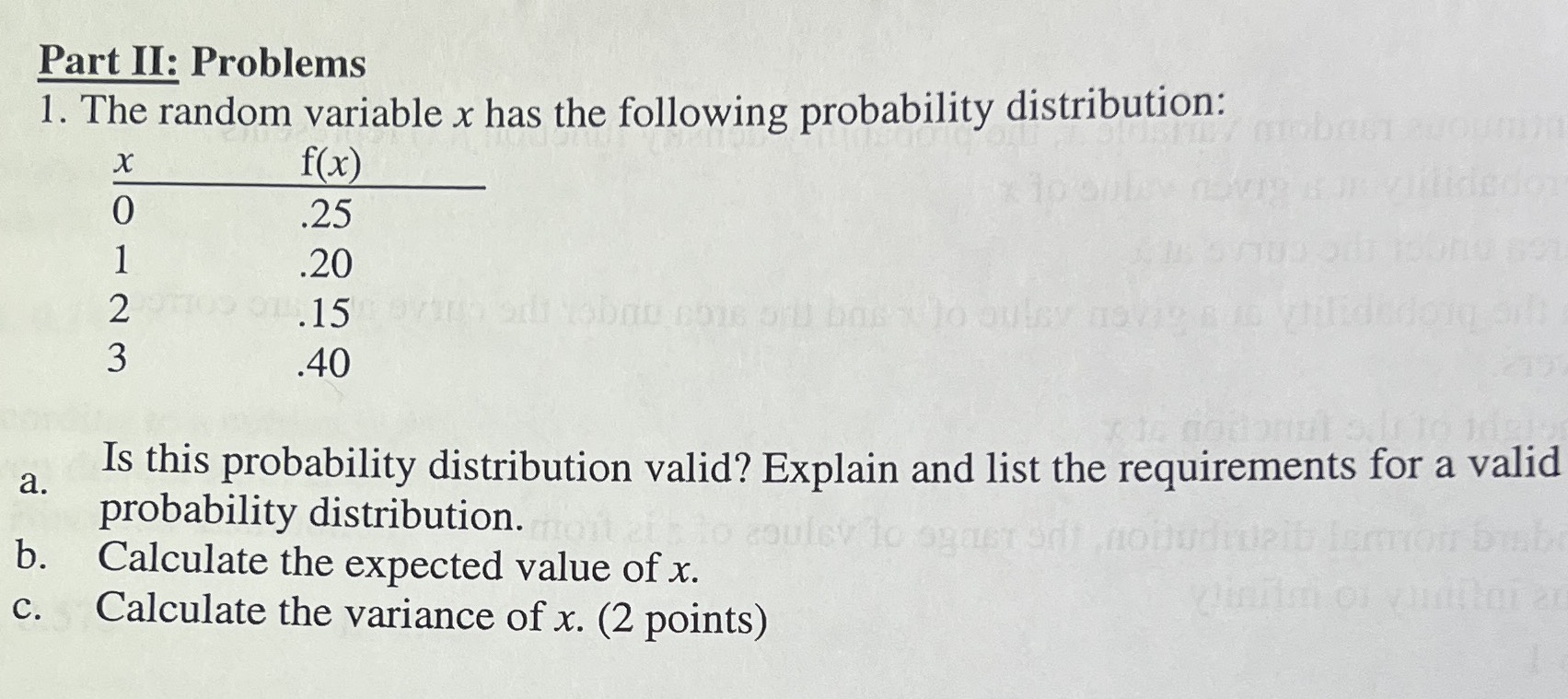### ¿Todavía tienes preguntas de matemáticas?

Pregunte a nuestros tutores expertos
Algebra
Pregunta1. The random variable $$x$$ has the following probability distribution:

$$f ( x )$$ a. Is this probability distribution valid? Explain and list the requirements for a valid probability distribution. c. Calculate the expected value of $$x$$ .

$$a.Yes,\ The\ sum \ of \ the \ probabilities \ is \ equal \ to 1\\b.1.7\\c.1.5894736842105$$